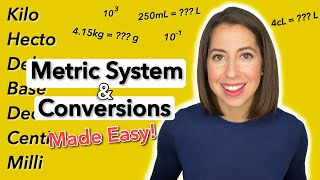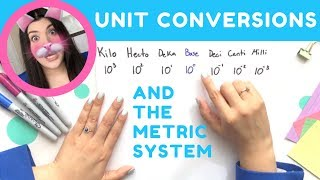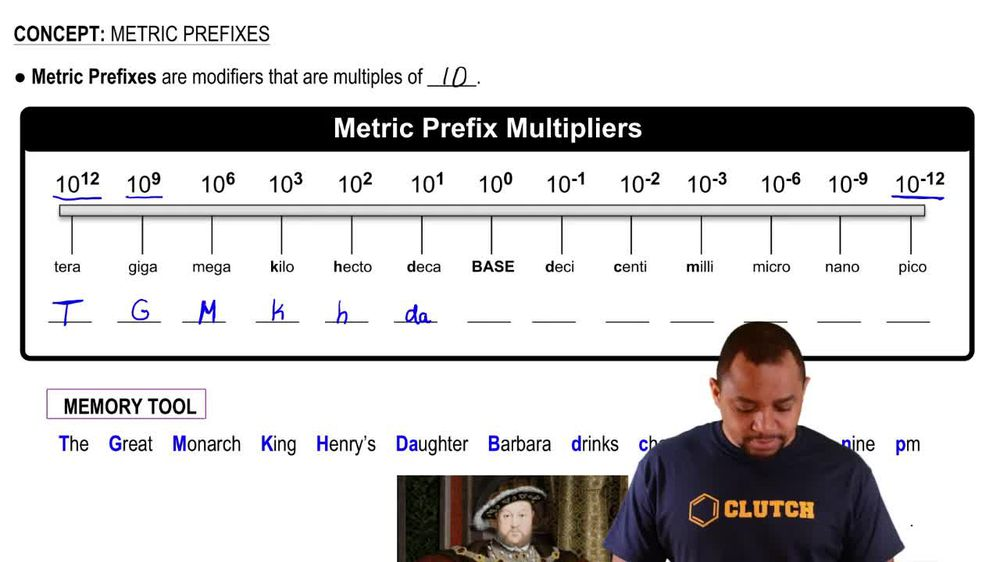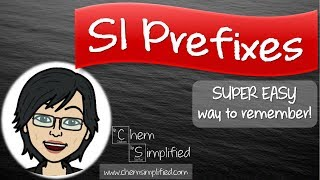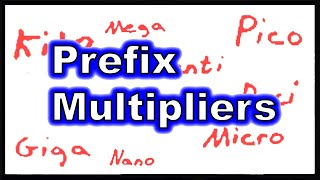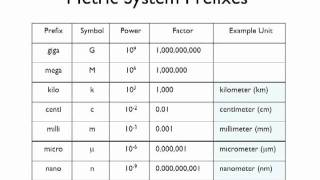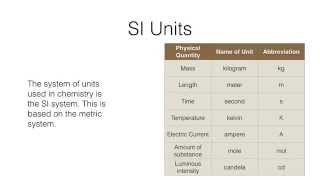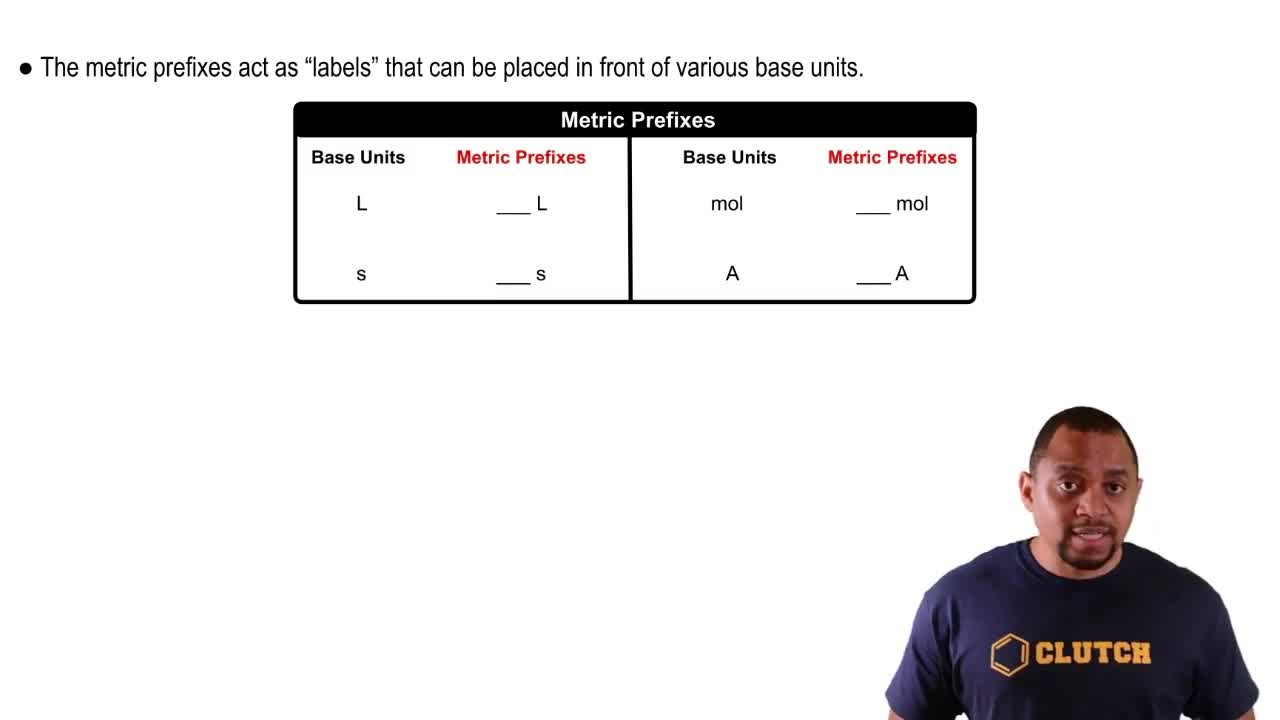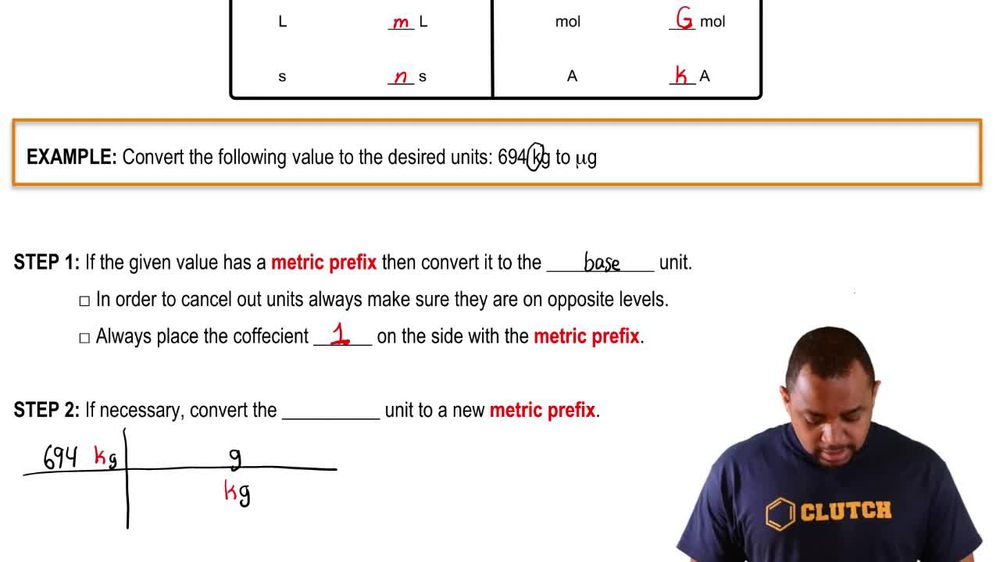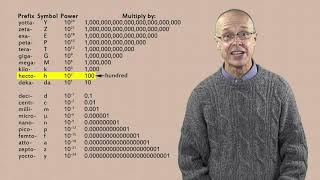Start typing, then use the up and down arrows to select an option from the list.
1. 1. Intro to General Chemistry2. Metric Prefixes
Problem

# Use the prefix multipliers to express each measurement without exponents. a. 1.2 * 10 - 9 m b. 22 * 10 - 15 s c. 1.5 * 109 g d. 3.5 * 106 L

Relevant Solution1m
Play a video:
Hi everyone here we have a question telling us without using exponents express the following measurements. Using the appropriate metric prefixes 8.561 times 10 to the negative four m squared. So we have to thank what squared equals negative for, and 10 to the - equals 10 to the negative second squared, So eight point times 10 to the negative fourth meter squared equals 8. centimeters squared. Because cm is 10 to the -2. So that is our final answer. Thank you for watching. Bye.